1. Which of the the following graphic device information is odd man out in the below figure?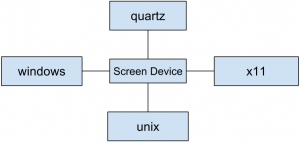a. quartz b. window c. unix d. x11

 2. Which of the following function has parameters shown in the below figure?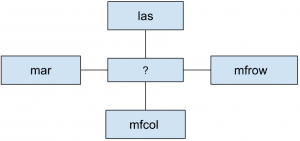a. par b. bar c. base d. all of the mentioned

 3. Which of the following block information is odd man out in the below figure?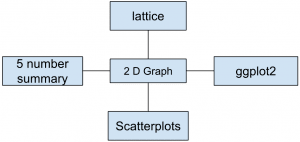a. Scatterplots b. 5 number summary c. 2D Graph d. None of the mentioned

 4. Which type of graph is shown in the following figure?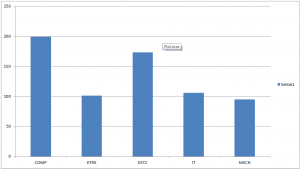a. Scatterplot b. Barplot c. Overlaying d. None of the mentioned

 5. Which of the following clustering type has characteristic shown in the below figure?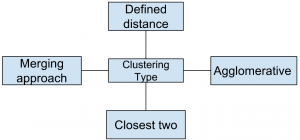a. Partitional b. Hierarchical c. Naive bayes d. None of the mentioned

 6. Which of the following graphs has properties in the below figure?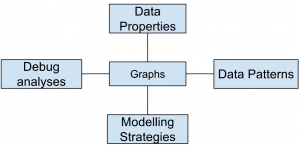a. Exploratory b. Inferential c. Causal d. None of the mentioned

 7. Which of the following dimension type graph is shown in the below figure?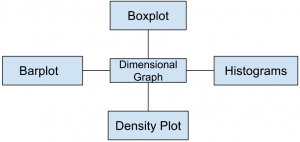a. one-dimensional b. two-dimensional c. three-dimensional d. none of the mentioned